## Selina Concise Mathematics Class 10 ICSE Solutions Chapter 20 Cylinder, Cone and Sphere (Surface Area and Volume) Ex 20D

These Solutions are part of Selina Concise Mathematics Class 10 ICSE Solutions. Here we have given Selina Concise Mathematics Class 10 ICSE Solutions Chapter 21 Trigonometrical Identities Ex 21D

Other Exercises

Question 1.
A solid sphere of radius 15 cm is melted and recast into solid right circular cones of radius 2.5 cm and height 8 cm. Calculate the number of cones recast.
Solution:Question 2.
A hollow sphere of internal and external diameters 4 cm and 8 cm respectively is melted into a cone of base diameter 8 cm. Find the height of the cone. 
Solution:
External diameter = 8cm
∴ Radius (R) = $$\frac { 8 }{ 2 }$$ = 4 cm
Internal diameter = 4 cm
∴ Radius (r) = $$\frac { 4 }{ 2 }$$ = 2cm.
Volume of metal used in hollow sphere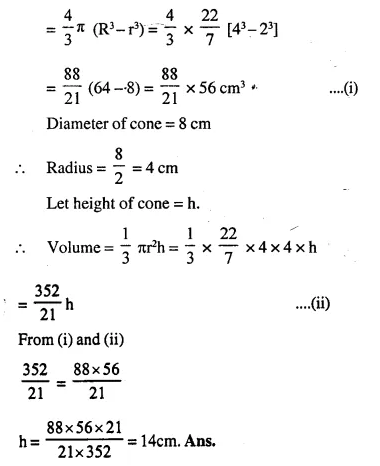Question 3.
The radii of the internal and external surfaces of a metallic spherical shell are 3 cm and 5 cm respectively. It is melted and recast into a solid right circular cone of height 32 cm. Find the diameter of the base of the cone.
Solution:
Inner radius of spherical shell (r) = 3 cm
and outer radius (R) = 5 cm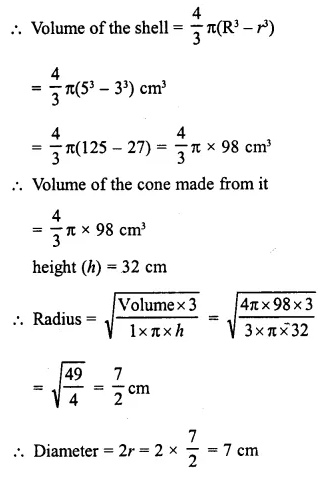Question 4.
Total volume of three identical cones is the same as that of a bigger cone whose height is 9 cm and diameter 40 cm. Find the radius of the base of each smaller cone, if height of each is 108 cm.
Solution:
Height of bigger cone (H) = 9 cm
Diameter 40 cm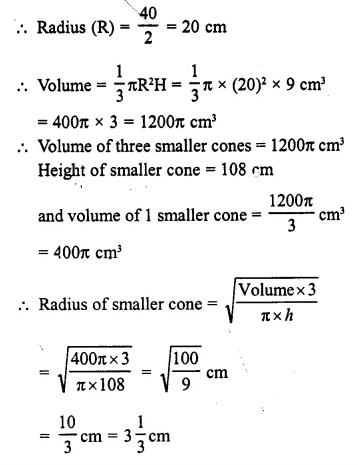Question 5.
A solid rectangular block of metal 49 cm by 44 cm by 18 cm is melted and formed into a solid sphere. Calculate the radius of the sphere.
Solution:
Dimensions of rectangular block of metal = 49cm x 44 cm x 18 cm.
∴ Volume = 49 x 44 x 18 cm3 = 38808 cm3
Let radius of solid sphere = r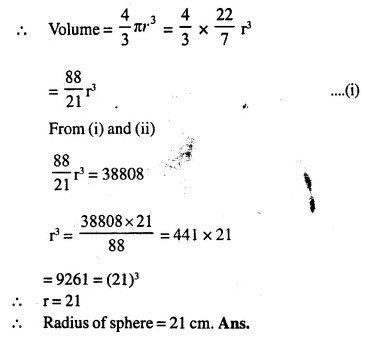Question 6.
A hemisphere bowl of internal radius 9 cm is full of liquid. This liquid is to be filled into conical shaped small containers each of diameter 3 cm and height 4 cm. How many containers are necessary to empty the bowl?
Solution:
Internal radius of hemispherical bowl (r) = 9 cm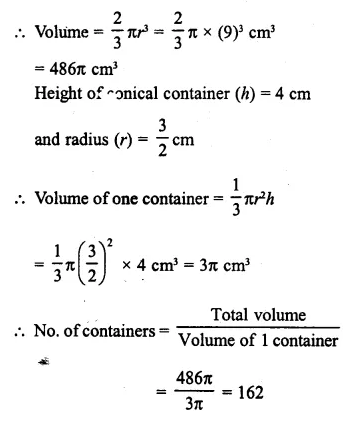Question 7.
A hemispherical bowl of diameter 7.2 cm is filled completely with chocolate sauce. This sauce is poured into an inverted cone of radius 4.8 cm. Find the height of the cone if it is completely filled. 
Solution:
Diameter of hemispherical bowl = 7.2 cmQuestion 8.
A solid cone of radius 5 cm and height 8 cm is melted and made into small spheres of radius 0.5 cm. Find the number of spheres formed. 
Solution:
Radius of solid cone = (r) = 5 cm
and height (h) = 8 cmQuestion 9.
The total area of a solid metallic sphere is 1256 cm2. It is melted and recast into solid right circular cones of radius 2.5 cm and height 8 cm. Calculate:,
(i)  the radius of the solid sphere,
(ii) the number of cones recast.   Take π = 3.14              
Solution:
Total area of solid sphere = 125Question 10.
A solid metallic cone, with radius 6 cm and height 10 cm, is made of some heavy metal A. In order to reduce its weight, a conical hole is made in the cone as shown and it is completely filled with a lighter metal B. The conical hole has a diameter of 6 cm and depth 4 cm. Calculate the ratio of the volume of metal A to the volume of the metal B in the solid.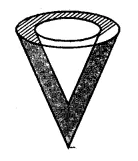Solution:
Radius of solid metallic cone A(R) = 6 cm
and height (H) = 10 cm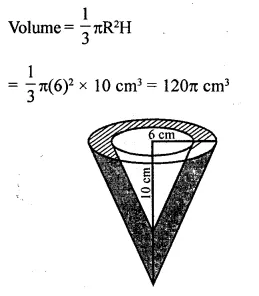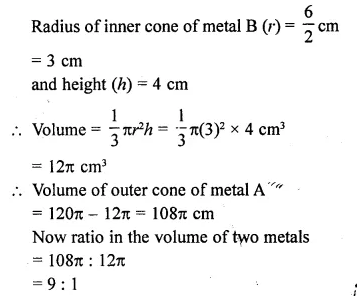Question 11.
A hollow sphere of internal and external radii 6 cm and 8 cm respectively is melted and recast into small cones of base radius 2 cm and height 8 cm. Find the number of cones.           
Solution:
Inner radius of a hollow sphere (r) = 6 cm
and outer radius (R) = 8 cm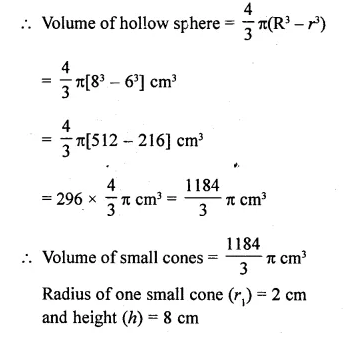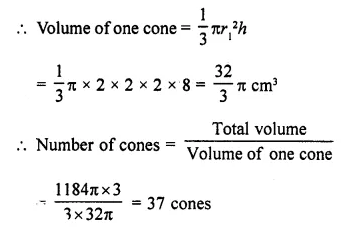Question 12.
The surface area of a solid metallic sphere is 2464 cm2. It is melted and recast into solid right circular cones of radius 3.5 cm and height 7 cm. Calculate:
(i) the radius of the sphere.
(ii) the number of cones recast. (Take π = 22/7)
Solution:
(i) Surface area=4πr2=2464 cm2 (given)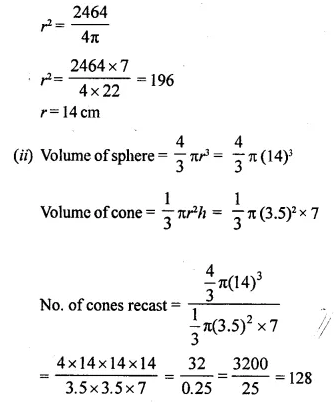Hope given Selina Concise Mathematics Class 10 ICSE Solutions Chapter 20 Cylinder, Cone and Sphere Ex 20D are helpful to complete your math homework.

If you have any doubts, please comment below. Learn Insta try to provide online math tutoring for you.